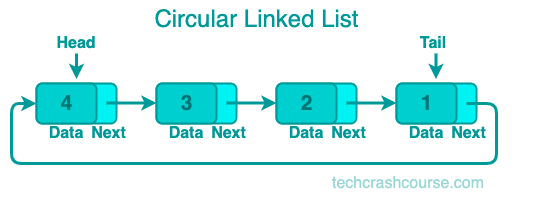A circular linked list is a data structure where each node contains a reference to the next node in the list, and the last node in the list points back to the first node. This creates a circular loop that can be traversed infinitely.

In a singly linked list, the last node points to NULL, but in a circular linked list, the last node points back to the first node.## Representation of a Circular Linked List

A circular linked list can be represented as a collection of nodes, where each node contains two fields: data and next. The data field contains the value of the node, and the next field contains a reference to the next node in the list. The last node in the list contains a reference to the first node, creating a circular loop.

### Uses of Circular Linked List

Circular linked lists are useful in situations where we need to traverse a list indefinitely, for example in a circular buffer or a playlist of songs that should loop indefinitely.

• A circular linked list can be traversed indefinitely.

• Insertion and deletion operations can be performed efficiently at any position in the list.

• It is more complex to implement than a singly linked list.

• It requires extra memory to store the reference from the last node back to the first node.

## Common Circular Linked List Operations

To add a node to a circular linked list, we need to create a new node and set its next field to point to the first node in the list. Then we set the next field of the last node in the list to point to the new node. Finally, we update the head pointer to point to the new node.

### Traverse a Circular Linked List

To traverse a circular linked list, we start at the head node and follow the next pointers until we reach the head node again.

### Delete a Node from Circular Linked List

To delete a node from a circular linked list, we need to update the next field of the previous node to point to the node after the one we want to delete. Then we can free the memory allocated to the node we want to delete. If we are deleting the first node in the list, we also need to update the head pointer.

### Search an Element in Circular Linked List

To search for an element in a circular linked list, we start at the head node and follow the next pointers until we find the node containing the element we are looking for, or we reach the head node again.

### Circular Linked List Operations Time Complexity

The time complexity of the common operations on a Singly Linked List are as follows:
• To add a node at the end of a circular linked list: O(1)
• Traverse a circular linked list: O(n)
• Delete a node from circular linked list: O(n)
• Search an element in circular linked list: O(n)

## Circular Linked List Implementation Program

Here's an example program that demonstrates how to implement a circular linked list in C.

```#include <stdio.h>
#include <stdlib.h>

// Node structure
struct node {
int data;
struct node* next;
};

struct node* new_node = (struct node*)malloc(sizeof(struct node));

new_node->data = new_data;

new_node->next = new_node;
printf("Node added : %d \n", new_data);
return;
}

last = last->next;
}

last->next = new_node;
printf("Node added : %d \n", new_data);
}

// Traverse the circular linked list

do {
printf("%d ", temp->data);
temp = temp->next;
printf("\n");
}

// Delete a node from the circular linked list
void deleteNode(struct node** head_ref, int key) {
return;
}

struct node* curr = *head_ref, *prev;

while (curr->data != key) {
return;
}
prev = curr;
curr = curr->next;
}

free(curr);
return;
}

prev = prev->next;
}
free(curr);
} else if (curr->next == *head_ref) {
free(curr);
} else {
prev->next = curr->next;
free(curr);
}

printf("Node deleted : %d \n", key);
}

// Search for an element in the circular linked list
int searchList(struct node* head, int key) {
int count = 0;

return -1;
}

do {
count++;
if (current->data == key) {
return count;
}
current = current->next;

return -1;
}

// Main function
int main() {

// Delete a node from the circular linked list

// Search for an element in the circular linked list
int key = 4;
if (pos == -1) {
} else {
printf("%d found at position %d\n", key, pos);
}

// Traverse the circular linked list

return 0;
}
```
Output
```Node added : 1